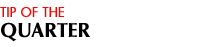The Contest ContinuesHave a suggestion for the newsletter?

We want to hear from you!
Call Your Customer Service Representative at
713-526-2111, or
e-mail us at total1dpnow@total1services.com

 In Our Next Issue: TOTAL/1 Staff Spotlight All About Imaging Credit Union Spotlight Your TOTAL/1 Questions - Answered! More How To Tips Contest Winners, Upcoming Events, and more!By Ann Verzwyvelt

Making a Loan Payment or Certificate Dividend Correction

When making a loan payment or certificate dividend correction, your system will prompt you to decide whether you want to accrue the interest reversal or whether you want to compute the accrued dividends. This step can be confusing, because the answer varies depending on the situation.

To help you decide how to answer, you will need to first manually calculate each loan payment or dividend for the interest period.

Below are step-by-step procedures for calculating loan interest, as well as procedures for manually calculating dividends, which can be used for shares or certificates.

Loan Interest Calculation Procedures
Multiply the loan balance by the interest rate, divide by 365 days, and multiply again by the number of days at that balance:

(Loan Balance x Interest Rate)
__________________________      X Number of Days at Balance
365 Days

Record the result of your calculation. Repeat the above for each period for which you are calculating interest.

Day In / Day Out Calculation Method (DIDO):
Using an account history printout, multiply the account balance by the dividend rate, divide by 365 days, and multiply again by the number of days that the account remains at that balance:

(Balance x Dividend Rate)
__________________________      X Number of Days at Balance
365 Days

Record the result of your calculation. Repeat the above for each time the balance changes within the dividend period.

Once you have run calculations for each balance change, add all of your results to determine the total amount of dividends due the account.

Average Daily Balance Calculation Method (ADB):
Using an account history printout, add each day's balance throughout t he dividend period, and obtain a total.

Divide this total by the number of days within your dividend period, giving the Average Daily Balance.

Multiply this calculation result by the dividend rate to determine the total amount of dividends due the account.

(Sum of Daily Balance in Dividend Period)
_______________________________________     X Dividend Rate
Number of Days in Dividend Period

Note: If the account was opened after the beginning of the dividend period, only calculate the number of days from the date of the initial deposit.

Both dividend and interest recognize the "effective date" of a transaction. Remember to consider the effect of back-dating on balances in your calculations.

Contact your Total/1 Customer Service Representative if you have any questions about these or any other procedures. We're here to help!

If you have any questions, contact your TOTAL/1 Customer Service Representative.